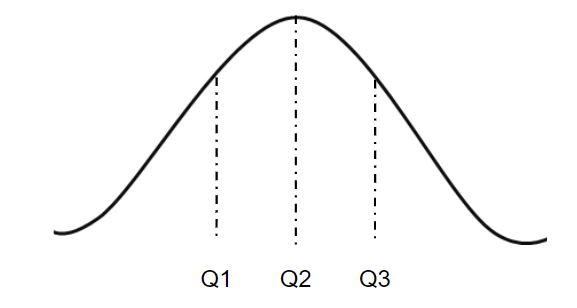GeeksforGeeks App
Open AppBrowser
Continue

# numpy.quantile() in Python

numpy.quantile(arr, q, axis = None) : Compute the qth quantile of the given data (array elements) along the specified axis. Quantile plays a very important role in Statistics when one deals with the Normal Distribution.In the figure given above, Q2 is the median of the normally distributed data. Q3 – Q2 represents the Interquartile Range of the given dataset.

Parameters : arr : [array_like]input array. q : quantile value. axis : [int or tuples of int]axis along which we want to calculate the quantile value. Otherwise, it will consider arr to be flattened(works on all the axis). axis = 0 means along the column and axis = 1 means working along the row. out : [ndarray, optional]Different array in which we want to place the result. The array must have same dimensions as expected output. Results : qth quantile of the array (a scalar value if axis is none) or array with quantile values along specified axis.

Code #1:

## Python3

 `# Python Program illustrating``# numpy.quantile() method``import` `numpy as np`  `# 1D array``arr ``=` `[``20``, ``2``, ``7``, ``1``, ``34``]` `print``("arr : ", arr)``print``("Q2 quantile of arr : ", np.quantile(arr, .``50``))``print``("Q1 quantile of arr : ", np.quantile(arr, .``25``))``print``("Q3 quantile of arr : ", np.quantile(arr, .``75``))``print``("``100th` `quantile of arr : ", np.quantile(arr, .``1``))``  `

Output :

```arr : [20, 2, 7, 1, 34]
Q2 quantile of arr : 7.0)
Q1 quantile of arr : 2.0)
Q3 quantile of arr : 20.0)
100th quantile of arr : 1.4)```

Code #2:

## Python3

 `# Python Program illustrating``# numpy.quantile() method``import` `numpy as np`` ` `# 2D array``arr ``=` `[[``14``, ``17``, ``12``, ``33``, ``44``], ``       ``[``15``, ``6``, ``27``, ``8``, ``19``],``       ``[``23``, ``2``, ``54``, ``1``, ``4``, ]]``print``("\narr : \n", arr)``   ` `# quantile of the flattened array``print``("\n50th quantile of arr, axis ``=` `None` `: ", np.quantile(arr, .``50``))``print``("``0th` `quantile of arr, axis ``=` `None` `: ", np.quantile(arr, ``0``))``   ` `# quantile along the axis = 0``print``("\n50th quantile of arr, axis ``=` `0` `: ", np.quantile(arr, .``25``, axis ``=` `0``))``print``("``0th` `quantile of arr, axis ``=` `0` `: ", np.quantile(arr, ``0``, axis ``=` `0``))``  ` `# quantile along the axis = 1``print``("\n50th quantile of arr, axis ``=` `1` `: ", np.quantile(arr, .``50``, axis ``=` `1``))``print``("``0th` `quantile of arr, axis ``=` `1` `: ", np.quantile(arr, ``0``, axis ``=` `1``))`` ` `print``("\n0th quantile of arr, axis ``=` `1` `: \n",``   ``np.quantile(arr, .``50``, axis ``=` `1``, keepdims ``=` `True``))``print``("\n0th quantile of arr, axis ``=` `1` `: \n",``   ``np.quantile(arr, ``0``, axis ``=` `1``, keepdims ``=` `True``))`

Output :

```arr :
[[14, 17, 12, 33, 44], [15, 6, 27, 8, 19], [23, 2, 54, 1, 4]]

50th quantile of arr, axis = None : 15.0
0th quantile of arr, axis = None : 1)

50th quantile of arr, axis = 0 : [14.5  4.  19.5  4.5 11.5]
0th quantile of arr, axis = 0 : [14  2 12  1  4]

50th quantile of arr, axis = 1 : [17. 15.  4.]
0th quantile of arr, axis = 1 : [12  6  1]

0th quantile of arr, axis = 1 :
[[17.]
[15.]
[ 4.]]

0th quantile of arr, axis = 1 :
[
[ 6]
[ 1]]```

My Personal Notes arrow_drop_up